# SymPy¶

SymPy is a package for symbolic calculations in python, similar to Mathematica. It works with expressions containing symbols.

In :
from sympy import *
init_printing()


Symbols are basic bricks used to construct expressions. Each symbol has a name used for printing expressions. Objects of the class Symbol should be created and assigned to python variables in order to be used in expressions. The symbol name and the name of the variable to which this symbol is assigned are two independent things, and one may write abc=Symbol('xyz'). But then one has to write abc in input expressions, while SymPy will write xyz in output ones, producing unnecessary confusion. The python variable name should better be the same as the symbol name.

In languages specifically designed for symbolic calculations, such as Mathematica, if a variable to which nothing has been assigned is used, it automatically means a symbol with the same name. Python has not been designed for symbolic calculations. If you use a variable to which nothing has been assigned, you will get an error message. Symbol objects have to be created explicitly.

In :
x=Symbol('x')

In :
a=x**2-1
a

Out:
$$x^{2} - 1$$

Several symbols can be defined at once. The string is split at spaces.

In :
y,z=symbols('y z')


Let's substitute $y+1$ for $x$.

In :
a.subs(x,y+1)

Out:
$$\left(y + 1\right)^{2} - 1$$

## Polynomials and rational functions¶

SymPy does not expand brackets automatically. The function expand is used for this.

In :
a=(x+y-z)**6
a

Out:
$$\left(x + y - z\right)^{6}$$
In :
a=expand(a)
a

Out:
$$x^{6} + 6 x^{5} y - 6 x^{5} z + 15 x^{4} y^{2} - 30 x^{4} y z + 15 x^{4} z^{2} + 20 x^{3} y^{3} - 60 x^{3} y^{2} z + 60 x^{3} y z^{2} - 20 x^{3} z^{3} + 15 x^{2} y^{4} - 60 x^{2} y^{3} z + 90 x^{2} y^{2} z^{2} - 60 x^{2} y z^{3} + 15 x^{2} z^{4} + 6 x y^{5} - 30 x y^{4} z + 60 x y^{3} z^{2} - 60 x y^{2} z^{3} + 30 x y z^{4} - 6 x z^{5} + y^{6} - 6 y^{5} z + 15 y^{4} z^{2} - 20 y^{3} z^{3} + 15 y^{2} z^{4} - 6 y z^{5} + z^{6}$$

Degree of the polynomial $a$ in $x$.

In :
degree(a,x)

Out:
$$6$$

Let's collect terms with same power of $x$ together.

In :
collect(a,x)

Out:
$$x^{6} + x^{5} \left(6 y - 6 z\right) + x^{4} \left(15 y^{2} - 30 y z + 15 z^{2}\right) + x^{3} \left(20 y^{3} - 60 y^{2} z + 60 y z^{2} - 20 z^{3}\right) + x^{2} \left(15 y^{4} - 60 y^{3} z + 90 y^{2} z^{2} - 60 y z^{3} + 15 z^{4}\right) + x \left(6 y^{5} - 30 y^{4} z + 60 y^{3} z^{2} - 60 y^{2} z^{3} + 30 y z^{4} - 6 z^{5}\right) + y^{6} - 6 y^{5} z + 15 y^{4} z^{2} - 20 y^{3} z^{3} + 15 y^{2} z^{4} - 6 y z^{5} + z^{6}$$

Any polynomial with integer coefficients can be factorized into polynomials with integer coefficients (which cannot be factorized further). There exist efficient algorithms to do this.

In :
a=factor(a)
a

Out:
$$\left(x + y - z\right)^{6}$$

SymPy does not automatically cancel ratios of polynomials by their greatest common divisor. The function cancel is used for this.

In :
a=(x**3-y**3)/(x**2-y**2)
a

Out:
$$\frac{x^{3} - y^{3}}{x^{2} - y^{2}}$$
In :
cancel(a)

Out:
$$\frac{x^{2} + x y + y^{2}}{x + y}$$

SymPy does not automatically bring sums of rational expressions to common denominator. The function together is used for this.

In :
a=y/(x-y)+x/(x+y)
a

Out:
$$\frac{x}{x + y} + \frac{y}{x - y}$$
In :
together(a)

Out:
$$\frac{x \left(x - y\right) + y \left(x + y\right)}{\left(x - y\right) \left(x + y\right)}$$

The function simplify tries to rewrite an expression in a simplest form. This concept is not well defined (different forms may be considered simplest in different contexts), and there exists no algorithm for such simplification. The function simplify works heuristically, and it is not possible to guess in advance what simplifications it will try. It is very convenient in interactive sessions in order to check if it will succeed in rewriting an expression in some reasonably good form. But it is not desirable to use it in programs. There one should better use more specialized functions which perform well defined expression transformations.

In :
simplify(a)

Out:
$$\frac{x^{2} + y^{2}}{x^{2} - y^{2}}$$

Partial fraction decomposition with respect to $x$.

In :
apart(a,x)

Out:
$$- \frac{y}{x + y} + \frac{y}{x - y} + 1$$

Let's substitute some values for the symbils $x$ and $y$.

In :
a=a.subs({x:1,y:2})
a

Out:
$$- \frac{5}{3}$$

And how much is it numerically?

In :
a.n()

Out:
$$-1.66666666666667$$

## Elementary functions¶

SymPy automatically applies simplifications of elementary functions which are correct everywhere.

In :
sin(-x)

Out:
$$- \sin{\left (x \right )}$$
In :
cos(pi/4),tan(5*pi/6)

Out:
$$\left ( \frac{\sqrt{2}}{2}, \quad - \frac{\sqrt{3}}{3}\right )$$

SymPy can work with floating point numbers having arbitrarily high precision. Here is $\pi$ with 100 significant digits.

In :
pi.n(100)

Out:
$$3.141592653589793238462643383279502884197169399375105820974944592307816406286208998628034825342117068$$

E is the base of natural logarithms.

In :
log(1),log(E)

Out:
$$\left ( 0, \quad 1\right )$$
In :
exp(log(x)),log(exp(x))

Out:
$$\left ( x, \quad \log{\left (e^{x} \right )}\right )$$

Why not $x$? Try $x=2\pi i$.

In :
sqrt(0)

Out:
$$0$$
In :
sqrt(x)**4,sqrt(x**4)

Out:
$$\left ( x^{2}, \quad \sqrt{x^{4}}\right )$$

Why not $x^2$? Try $x=i$.

Symbols can have certain properties. E.g., they can be positive. Then SymPy can simplify square roots better.

In :
p,q=symbols('p q',positive=True)
sqrt(p**2)

Out:
$$p$$
In :
sqrt(12*x**2*y),sqrt(12*p**2*y)

Out:
$$\left ( 2 \sqrt{3} \sqrt{x^{2} y}, \quad 2 \sqrt{3} p \sqrt{y}\right )$$

Let the symbol $n$ be integer (I is the imaginary unit).

In :
n=Symbol('n',integer=True)
exp(2*pi*I*n)

Out:
$$1$$

The method rewrite tries to rewrite an expression in terms of a given function.

In :
cos(x).rewrite(exp),exp(I*x).rewrite(cos)

Out:
$$\left ( \frac{e^{i x}}{2} + \frac{1}{2} e^{- i x}, \quad i \sin{\left (x \right )} + \cos{\left (x \right )}\right )$$
In :
asin(x).rewrite(log)

Out:
$$- i \log{\left (i x + \sqrt{- x^{2} + 1} \right )}$$

The function trigsimp tries to rewrite a trigonometric expression in a simplest form. In programs it is better to use more specialized functions.

In :
trigsimp(2*sin(x)**2+3*cos(x)**2)

Out:
$$\cos^{2}{\left (x \right )} + 2$$

The function expand_trig expands sines and cosines of sums and multiple angles.

In :
expand_trig(sin(x-y)),expand_trig(sin(2*x))

Out:
$$\left ( \sin{\left (x \right )} \cos{\left (y \right )} - \sin{\left (y \right )} \cos{\left (x \right )}, \quad 2 \sin{\left (x \right )} \cos{\left (x \right )}\right )$$

The inverse transformation, rewriting products and powers of sines and cosines into expressions linear in these functions, is needed more often. Suppose we work with a truncated Fourier series.

In :
a1,a2,b1,b2=symbols('a1 a2 b1 b2')
a=a1*cos(x)+a2*cos(2*x)+b1*sin(x)+b2*sin(2*x)
a

Out:
$$a_{1} \cos{\left (x \right )} + a_{2} \cos{\left (2 x \right )} + b_{1} \sin{\left (x \right )} + b_{2} \sin{\left (2 x \right )}$$

We want to square it and get a truncated Fourier series again.

In :
a=(a**2).rewrite(exp).expand().rewrite(cos).expand()
a

Out:
$$\frac{a_{1}^{2}}{2} \cos{\left (2 x \right )} + \frac{a_{1}^{2}}{2} + a_{1} a_{2} \cos{\left (x \right )} + a_{1} a_{2} \cos{\left (3 x \right )} + a_{1} b_{1} \sin{\left (2 x \right )} + a_{1} b_{2} \sin{\left (x \right )} + a_{1} b_{2} \sin{\left (3 x \right )} + \frac{a_{2}^{2}}{2} \cos{\left (4 x \right )} + \frac{a_{2}^{2}}{2} - a_{2} b_{1} \sin{\left (x \right )} + a_{2} b_{1} \sin{\left (3 x \right )} + a_{2} b_{2} \sin{\left (4 x \right )} - \frac{b_{1}^{2}}{2} \cos{\left (2 x \right )} + \frac{b_{1}^{2}}{2} + b_{1} b_{2} \cos{\left (x \right )} - b_{1} b_{2} \cos{\left (3 x \right )} - \frac{b_{2}^{2}}{2} \cos{\left (4 x \right )} + \frac{b_{2}^{2}}{2}$$
In :
a.collect([cos(x),cos(2*x),cos(3*x),sin(x),sin(2*x),sin(3*x)])

Out:
$$\frac{a_{1}^{2}}{2} + a_{1} b_{1} \sin{\left (2 x \right )} + \frac{a_{2}^{2}}{2} \cos{\left (4 x \right )} + \frac{a_{2}^{2}}{2} + a_{2} b_{2} \sin{\left (4 x \right )} + \frac{b_{1}^{2}}{2} - \frac{b_{2}^{2}}{2} \cos{\left (4 x \right )} + \frac{b_{2}^{2}}{2} + \left(\frac{a_{1}^{2}}{2} - \frac{b_{1}^{2}}{2}\right) \cos{\left (2 x \right )} + \left(a_{1} a_{2} - b_{1} b_{2}\right) \cos{\left (3 x \right )} + \left(a_{1} a_{2} + b_{1} b_{2}\right) \cos{\left (x \right )} + \left(a_{1} b_{2} - a_{2} b_{1}\right) \sin{\left (x \right )} + \left(a_{1} b_{2} + a_{2} b_{1}\right) \sin{\left (3 x \right )}$$

The function expand_log transforms logarithms of products and powers (of positive quantities) into sums of logarithms; logcombine performs the inverse transformation.

In :
a=expand_log(log(p*q**2))
a

Out:
$$\log{\left (p \right )} + 2 \log{\left (q \right )}$$
In :
logcombine(a)

Out:
$$\log{\left (p q^{2} \right )}$$

The function expand_power_exp rewrites powers whose exponents are sums via products of powers.

In :
expand_power_exp(x**(p+q))

Out:
$$x^{p} x^{q}$$

The function expand_power_base rewrites powers whose bases are products via products of powers.

In :
expand_power_base((x*y)**n)

Out:
$$x^{n} y^{n}$$

The function powsimp performs the inverse transformations.

In :
powsimp(exp(x)*exp(2*y)),powsimp(x**n*y**n)

Out:
$$\left ( e^{x + 2 y}, \quad \left(x y\right)^{n}\right )$$

New symbolic functions can be introduced. They may have an arbitrary numbers of arguments.

In :
f=Function('f')
f(x)+f(x,y)

Out:
$$f{\left (x \right )} + f{\left (x,y \right )}$$

## Expression structure¶

Internally expressions are are trees. The function srepr returns a string representing this tree.

In :
srepr(x+1)

Out:
"Add(Symbol('x'), Integer(1))"
In :
srepr(x-1)

Out:
"Add(Symbol('x'), Integer(-1))"
In :
srepr(x-y)

Out:
"Add(Symbol('x'), Mul(Integer(-1), Symbol('y')))"
In :
srepr(2*x*y/3)

Out:
"Mul(Rational(2, 3), Symbol('x'), Symbol('y'))"
In :
srepr(x/y)

Out:
"Mul(Symbol('x'), Pow(Symbol('y'), Integer(-1)))"

One may use the functions Add, Mul, Pow, etc. instead of the binary operations +, *, **, etc.

In :
Mul(x,Pow(y,-1))

Out:
$$\frac{x}{y}$$
In :
srepr(f(x,y))

Out:
"Function('f')(Symbol('x'), Symbol('y'))"

The attribute func is the top-level function of an expression, and args is the list of its agruments.

In :
a=2*x*y**2
a.func

Out:
sympy.core.mul.Mul
In :
a.args

Out:
$$\left ( 2, \quad x, \quad y^{2}\right )$$
In :
for i in a.args:
print(i)

2
x
y**2


The function subs substitutes an expression for a symbol.

In :
a.subs(y,2)

Out:
$$8 x$$

It can perform substitutions for several symbols. To this end, one calls it with a list of tuples or a dictionary.

In :
a.subs([(x,pi),(y,2)])

Out:
$$8 \pi$$
In :
a.subs({x:pi,y:2})

Out:
$$8 \pi$$

It can substitute not only for a symbol but also for a subexpression - a function with arguments.

In :
a=f(x)+f(y)
a.subs(f(y),1)

Out:
$$f{\left (x \right )} + 1$$
In :
(2*x*y*z).subs(x*y,z)

Out:
$$2 z^{2}$$
In :
(x+x**2+x**3+x**4).subs(x**2,y)

Out:
$$x^{3} + x + y^{2} + y$$

Substitutions are performed sequentially. In this case, first $x$ is replaced by $y$ producing $y^3+y^2$; then $y$ is replaced by $x$ in this result.

In :
a=x**2+y**3
a.subs([(x,y),(y,x)])

Out:
$$x^{3} + x^{2}$$

Interchanging these substitutions leads to a different result.

In :
a.subs([(y,x),(x,y)])

Out:
$$y^{3} + y^{2}$$

But if one calls subs with the keyword parameter simultaneous=True, all substitutions are preformed simultaneously. In this way one can, e.g., interchange $x$ and $y$.

In :
a.subs([(x,y),(y,x)],simultaneous=True)

Out:
$$x^{3} + y^{2}$$

A function can be replaced by another function.

In :
g=Function('g')
a=f(x)+f(y)
a.subs(f,g)

Out:
$$g{\left (x \right )} + g{\left (y \right )}$$

The method replace searches for subexpressions matching a pattern (with wildcards) and replaces them by a given expression.

In :
a=Wild('a')
(f(x)+f(x+y)).replace(f(a),a**2)

Out:
$$x^{2} + \left(x + y\right)^{2}$$
In :
(f(x,x)+f(x,y)).replace(f(a,a),a**2)

Out:
$$x^{2} + f{\left (x,y \right )}$$
In :
a=x**2+y**2
a.replace(x,x+1)

Out:
$$y^{2} + \left(x + 1\right)^{2}$$

Only a complete subtree can match a pattern, not a subset of factors in a product or a smaller power in a larger one.

In :
a=2*x*y*z
a.replace(x*y,z)

Out:
$$2 x y z$$
In :
(x+x**2+x**3+x**4).replace(x**2,y)

Out:
$$x^{4} + x^{3} + x + y$$

## Solving equations¶

In :
a,b,c,d,e,f=symbols('a b c d e f')


An equation is represented by the function Eq with two arguments. The function solve returns a list of solutions.

In :
solve(Eq(a*x,b),x)

Out:
$$\left [ \frac{b}{a}\right ]$$

Instead of equations, one may pass just expressions to solve; they mean equations <expression>=0.

In :
solve(a*x+b,x)

Out:
$$\left [ - \frac{b}{a}\right ]$$

A square equation has 2 solutions.

In :
solve(a*x**2+b*x+c,x)

Out:
$$\left [ \frac{1}{2 a} \left(- b + \sqrt{- 4 a c + b^{2}}\right), \quad - \frac{1}{2 a} \left(b + \sqrt{- 4 a c + b^{2}}\right)\right ]$$

A system of linear equations.

In :
solve([a*x+b*y-e,c*x+d*y-f],[x,y])

Out:
$$\left \{ x : \frac{- b f + d e}{a d - b c}, \quad y : \frac{a f - c e}{a d - b c}\right \}$$

The function roots returns roots of a polynomial together with their multiplicities.

In :
roots(x**3-3*x+2,x)

Out:
$$\left \{ -2 : 1, \quad 1 : 2\right \}$$

The function solve_poly_system solves systems of polynomial equations by constructing their Gröbner bases.

In :
p1=x**2+y**2-1
p2=4*x*y-1
solve_poly_system([p1,p2],x,y)

Out:
$$\left [ \left ( 4 \left(-1 - \sqrt{- \frac{\sqrt{3}}{4} + \frac{1}{2}}\right) \sqrt{- \frac{\sqrt{3}}{4} + \frac{1}{2}} \left(- \sqrt{- \frac{\sqrt{3}}{4} + \frac{1}{2}} + 1\right), \quad - \sqrt{- \frac{\sqrt{3}}{4} + \frac{1}{2}}\right ), \quad \left ( - 4 \left(-1 + \sqrt{- \frac{\sqrt{3}}{4} + \frac{1}{2}}\right) \sqrt{- \frac{\sqrt{3}}{4} + \frac{1}{2}} \left(\sqrt{- \frac{\sqrt{3}}{4} + \frac{1}{2}} + 1\right), \quad \sqrt{- \frac{\sqrt{3}}{4} + \frac{1}{2}}\right ), \quad \left ( 4 \left(-1 - \sqrt{\frac{\sqrt{3}}{4} + \frac{1}{2}}\right) \sqrt{\frac{\sqrt{3}}{4} + \frac{1}{2}} \left(- \sqrt{\frac{\sqrt{3}}{4} + \frac{1}{2}} + 1\right), \quad - \sqrt{\frac{\sqrt{3}}{4} + \frac{1}{2}}\right ), \quad \left ( - 4 \left(-1 + \sqrt{\frac{\sqrt{3}}{4} + \frac{1}{2}}\right) \sqrt{\frac{\sqrt{3}}{4} + \frac{1}{2}} \left(\sqrt{\frac{\sqrt{3}}{4} + \frac{1}{2}} + 1\right), \quad \sqrt{\frac{\sqrt{3}}{4} + \frac{1}{2}}\right )\right ]$$

## Series¶

In :
exp(x).series(x,0,5)

Out:
$$1 + x + \frac{x^{2}}{2} + \frac{x^{3}}{6} + \frac{x^{4}}{24} + \mathcal{O}\left(x^{5}\right)$$

A series can start from a negative power.

In :
cot(x).series(x,n=5)

Out:
$$\frac{1}{x} - \frac{x}{3} - \frac{x^{3}}{45} + \mathcal{O}\left(x^{5}\right)$$

And even run over half-integer powers.

In :
sqrt(x*(1-x)).series(x,n=5)

Out:
$$\sqrt{x} - \frac{x^{\frac{3}{2}}}{2} - \frac{x^{\frac{5}{2}}}{8} - \frac{x^{\frac{7}{2}}}{16} - \frac{5 x^{\frac{9}{2}}}{128} + \mathcal{O}\left(x^{5}\right)$$
In :
log(gamma(1+x)).series(x,n=6).rewrite(zeta)

Out:
$$- \gamma x + \frac{\pi^{2} x^{2}}{12} - \frac{x^{3} \zeta\left(3\right)}{3} + \frac{\pi^{4} x^{4}}{360} - \frac{x^{5} \zeta\left(5\right)}{5} + \mathcal{O}\left(x^{6}\right)$$

Let's prepare 3 series.

In :
sinx=series(sin(x),x,0,8)
sinx

Out:
$$x - \frac{x^{3}}{6} + \frac{x^{5}}{120} - \frac{x^{7}}{5040} + \mathcal{O}\left(x^{8}\right)$$
In :
cosx=series(cos(x),x,n=8)
cosx

Out:
$$1 - \frac{x^{2}}{2} + \frac{x^{4}}{24} - \frac{x^{6}}{720} + \mathcal{O}\left(x^{8}\right)$$
In :
tanx=series(tan(x),x,n=8)
tanx

Out:
$$x + \frac{x^{3}}{3} + \frac{2 x^{5}}{15} + \frac{17 x^{7}}{315} + \mathcal{O}\left(x^{8}\right)$$

Products and ratios of series are not calculated automatically, the function series should be applied to them.

In :
series(tanx*cosx,n=8)

Out:
$$x - \frac{x^{3}}{6} + \frac{x^{5}}{120} - \frac{x^{7}}{5040} + \mathcal{O}\left(x^{8}\right)$$
In :
series(sinx/cosx,n=8)

Out:
$$x + \frac{x^{3}}{3} + \frac{2 x^{5}}{15} + \frac{17 x^{7}}{315} + \mathcal{O}\left(x^{8}\right)$$

And this series should be equal to 1. But since sinx and cosx are known only with a limited accuracy, we obtain 1 with the same accuracy.

In :
series(sinx**2+cosx**2,n=8)

Out:
$$1 + \mathcal{O}\left(x^{8}\right)$$

Here the leading terms have canceled, and the result can be obtained only with a lower accuracy.

In :
series((1-cosx)/x**2,n=6)

Out:
$$\frac{1}{2} - \frac{x^{2}}{24} + \frac{x^{4}}{720} + \mathcal{O}\left(x^{6}\right)$$

Series can be differentiated and integrated.

In :
diff(sinx,x)

Out:
$$1 - \frac{x^{2}}{2} + \frac{x^{4}}{24} - \frac{x^{6}}{720} + \mathcal{O}\left(x^{7}\right)$$
In :
integrate(cosx,x)

Out:
$$x - \frac{x^{3}}{6} + \frac{x^{5}}{120} - \frac{x^{7}}{5040} + \mathcal{O}\left(x^{9}\right)$$

A series (starting from a small term) can be substituted for an expansion variable in another series. Here are $\sin(\tan(x))$ and $\tan(\sin(x))$.

In :
st=series(sinx.subs(x,tanx),n=8)
st

Out:
$$x + \frac{x^{3}}{6} - \frac{x^{5}}{40} - \frac{55 x^{7}}{1008} + \mathcal{O}\left(x^{8}\right)$$
In :
ts=series(tanx.subs(x,sinx),n=8)
ts

Out:
$$x + \frac{x^{3}}{6} - \frac{x^{5}}{40} - \frac{107 x^{7}}{5040} + \mathcal{O}\left(x^{8}\right)$$
In :
series(ts-st,n=8)

Out:
$$\frac{x^{7}}{30} + \mathcal{O}\left(x^{8}\right)$$

It is not possible to substitute a numerical value for the expansion variable in a series (and hence to plot it). To this end one has to remove the $\mathcal{O}$ term first, transforming a series into a polynomial.

In :
sinx.removeO()

Out:
$$- \frac{x^{7}}{5040} + \frac{x^{5}}{120} - \frac{x^{3}}{6} + x$$

## Derivatives¶

In :
a=x*sin(x+y)
diff(a,x)

Out:
$$x \cos{\left (x + y \right )} + \sin{\left (x + y \right )}$$
In :
diff(a,y)

Out:
$$x \cos{\left (x + y \right )}$$

The second derivative in $x$ and the first one in $y$.

In :
diff(a,x,2,y)

Out:
$$- x \cos{\left (x + y \right )} + 2 \sin{\left (x + y \right )}$$

Expressions with undefined functions can be differentiated.

In :
a=x*f(x**2)
b=diff(a,x)
b

Out:
$$2 x^{2} \left. \frac{d}{d \xi_{1}} f{\left (\xi_{1} \right )} \right|_{\substack{ \xi_{1}=x^{2} }} + f{\left (x^{2} \right )}$$

What's this?

In :
print(b)

2*x**2*Subs(Derivative(f(_xi_1), _xi_1), (_xi_1,), (x**2,)) + f(x**2)


The function Derivative represents an unevaluated derivative. It can be evaluated by the method doit.

In :
a=Derivative(sin(x),x)
Eq(a,a.doit())

Out:
$$\frac{d}{d x} \sin{\left (x \right )} = \cos{\left (x \right )}$$

## Integrals¶

In :
integrate(1/(x*(x**2-2)**2),x)

Out:
$$\frac{1}{4} \log{\left (x \right )} - \frac{1}{8} \log{\left (x^{2} - 2 \right )} - \frac{1}{4 x^{2} - 8}$$
In :
integrate(1/(exp(x)+1),x)

Out:
$$x - \log{\left (e^{x} + 1 \right )}$$
In :
integrate(log(x),x)

Out:
$$x \log{\left (x \right )} - x$$
In :
integrate(x*sin(x),x)

Out:
$$- x \cos{\left (x \right )} + \sin{\left (x \right )}$$
In :
integrate(x*exp(-x**2),x)

Out:
$$- \frac{e^{- x^{2}}}{2}$$
In :
a=integrate(x**x,x)
a

Out:
$$\int x^{x}\, dx$$

This is an unevaluated integral.

In :
print(a)

Integral(x**x, x)

In :
a=Integral(sin(x),x)
Eq(a,a.doit())

Out:
$$\int \sin{\left (x \right )}\, dx = - \cos{\left (x \right )}$$

Definite integrals.

In :
integrate(sin(x),(x,0,pi))

Out:
$$2$$

oo means $\infty$.

In :
integrate(exp(-x**2),(x,0,oo))

Out:
$$\frac{\sqrt{\pi}}{2}$$
In :
integrate(log(x)/(1-x),(x,0,1))

Out:
$$- \frac{\pi^{2}}{6}$$

## Summing series¶

In :
summation(1/n**2,(n,1,oo))

Out:
$$\frac{\pi^{2}}{6}$$
In :
summation((-1)**n/n**2,(n,1,oo))

Out:
$$- \frac{\pi^{2}}{12}$$
In :
summation(1/n**4,(n,1,oo))

Out:
$$\frac{\pi^{4}}{90}$$

An unevaluated sum is denoted Sum.

In :
a=Sum(x**n/factorial(n),(n,0,oo))
Eq(a,a.doit())

Out:
$$\sum_{n=0}^{\infty} \frac{x^{n}}{n!} = e^{x}$$

## Limits¶

In :
limit((tan(sin(x))-sin(tan(x)))/x**7,x,0)

Out:
$$\frac{1}{30}$$

This limit is easy: just expand the numerator and the denominator into series. Things become more difficult if $x=0$ is an essential singularity. Let's calculate one-sided limits.

In :
limit((tan(sin(x))-sin(tan(x)))/(x**7+exp(-1/x)),x,0,'+')

Out:
$$\frac{1}{30}$$
In :
limit((tan(sin(x))-sin(tan(x)))/(x**7+exp(-1/x)),x,0,'-')

Out:
$$0$$

## Differential equations¶

In :
t=Symbol('t')
x=Function('x')
p=Function('p')


First order.

In :
dsolve(diff(x(t),t)+x(t),x(t))

Out:
$$x{\left (t \right )} = C_{1} e^{- t}$$

Second order.

In :
dsolve(diff(x(t),t,2)+x(t),x(t))

Out:
$$x{\left (t \right )} = C_{1} \sin{\left (t \right )} + C_{2} \cos{\left (t \right )}$$

A system of first-order equations.

In :
dsolve((diff(x(t),t)-p(t),diff(p(t),t)+x(t)))

Out:
$$\left [ x{\left (t \right )} = C_{1} \sin{\left (t \right )} + C_{2} \cos{\left (t \right )}, \quad p{\left (t \right )} = C_{1} \cos{\left (t \right )} - C_{2} \sin{\left (t \right )}\right ]$$

## Linear algebra¶

In :
a,b,c,d,e,f=symbols('a b c d e f')


A matrix can be constructed from a list of lists.

In :
M=Matrix([[a,b,c],[d,e,f]])
M

Out:
$$\left[\begin{matrix}a & b & c\\d & e & f\end{matrix}\right]$$
In :
M.shape

Out:
$$\left ( 2, \quad 3\right )$$

A row matrix.

In :
Matrix([[1,2,3]])

Out:
$$\left[\begin{matrix}1 & 2 & 3\end{matrix}\right]$$

A column matrix.

In :
Matrix([1,2,3])

Out:
$$\left[\begin{matrix}1\\2\\3\end{matrix}\right]$$

A matrix can be constructed from a function.

In :
def g(i,j):
return Rational(1,i+j+1)
Matrix(3,3,g)

Out:
$$\left[\begin{matrix}1 & \frac{1}{2} & \frac{1}{3}\\\frac{1}{2} & \frac{1}{3} & \frac{1}{4}\\\frac{1}{3} & \frac{1}{4} & \frac{1}{5}\end{matrix}\right]$$

Or from an undefined function.

In :
g=Function('g')
M=Matrix(3,3,g)
M

Out:
$$\left[\begin{matrix}g{\left (0,0 \right )} & g{\left (0,1 \right )} & g{\left (0,2 \right )}\\g{\left (1,0 \right )} & g{\left (1,1 \right )} & g{\left (1,2 \right )}\\g{\left (2,0 \right )} & g{\left (2,1 \right )} & g{\left (2,2 \right )}\end{matrix}\right]$$
In :
M[1,2]

Out:
$$g{\left (1,2 \right )}$$
In :
M[1,2]=0
M

Out:
$$\left[\begin{matrix}g{\left (0,0 \right )} & g{\left (0,1 \right )} & g{\left (0,2 \right )}\\g{\left (1,0 \right )} & g{\left (1,1 \right )} & 0\\g{\left (2,0 \right )} & g{\left (2,1 \right )} & g{\left (2,2 \right )}\end{matrix}\right]$$
In :
M[2,:]

Out:
$$\left[\begin{matrix}g{\left (2,0 \right )} & g{\left (2,1 \right )} & g{\left (2,2 \right )}\end{matrix}\right]$$
In :
M[:,1]

Out:
$$\left[\begin{matrix}g{\left (0,1 \right )}\\g{\left (1,1 \right )}\\g{\left (2,1 \right )}\end{matrix}\right]$$
In :
M[0:2,1:3]

Out:
$$\left[\begin{matrix}g{\left (0,1 \right )} & g{\left (0,2 \right )}\\g{\left (1,1 \right )} & 0\end{matrix}\right]$$

A unit matrix.

In :
eye(3)

Out:
$$\left[\begin{matrix}1 & 0 & 0\\0 & 1 & 0\\0 & 0 & 1\end{matrix}\right]$$

A zero matrix.

In :
zeros(3)

Out:
$$\left[\begin{matrix}0 & 0 & 0\\0 & 0 & 0\\0 & 0 & 0\end{matrix}\right]$$
In :
zeros(2,3)

Out:
$$\left[\begin{matrix}0 & 0 & 0\\0 & 0 & 0\end{matrix}\right]$$

A diagonal matrix.

In :
diag(1,2,3)

Out:
$$\left[\begin{matrix}1 & 0 & 0\\0 & 2 & 0\\0 & 0 & 3\end{matrix}\right]$$
In :
M=Matrix([[a,1],[0,a]])
diag(1,M,2)

Out:
$$\left[\begin{matrix}1 & 0 & 0 & 0\\0 & a & 1 & 0\\0 & 0 & a & 0\\0 & 0 & 0 & 2\end{matrix}\right]$$

Operations with matrices.

In :
A=Matrix([[a,b],[c,d]])
B=Matrix([[1,2],[3,4]])
A+B

Out:
$$\left[\begin{matrix}a + 1 & b + 2\\c + 3 & d + 4\end{matrix}\right]$$
In :
A*B,B*A

Out:
$$\left ( \left[\begin{matrix}a + 3 b & 2 a + 4 b\\c + 3 d & 2 c + 4 d\end{matrix}\right], \quad \left[\begin{matrix}a + 2 c & b + 2 d\\3 a + 4 c & 3 b + 4 d\end{matrix}\right]\right )$$
In :
A*B-B*A

Out:
$$\left[\begin{matrix}3 b - 2 c & 2 a + 3 b - 2 d\\- 3 a - 3 c + 3 d & - 3 b + 2 c\end{matrix}\right]$$
In :
simplify(A**(-1))

Out:
$$\left[\begin{matrix}\frac{d}{a d - b c} & - \frac{b}{a d - b c}\\- \frac{c}{a d - b c} & \frac{a}{a d - b c}\end{matrix}\right]$$
In :
det(A)

Out:
$$a d - b c$$

### Eigenvalues and eigenvectors¶

In :
x=Symbol('x',real=True)

In :
M=Matrix([[(1-x)**3*(3+x),4*x*(1-x**2),-2*(1-x**2)*(3-x)],
[4*x*(1-x**2),-(1+x)**3*(3-x),2*(1-x**2)*(3+x)],
[-2*(1-x**2)*(3-x),2*(1-x**2)*(3+x),16*x]])
M

Out:
$$\left[\begin{matrix}\left(- x + 1\right)^{3} \left(x + 3\right) & 4 x \left(- x^{2} + 1\right) & \left(- x + 3\right) \left(2 x^{2} - 2\right)\\4 x \left(- x^{2} + 1\right) & - \left(- x + 3\right) \left(x + 1\right)^{3} & \left(x + 3\right) \left(- 2 x^{2} + 2\right)\\\left(- x + 3\right) \left(2 x^{2} - 2\right) & \left(x + 3\right) \left(- 2 x^{2} + 2\right) & 16 x\end{matrix}\right]$$
In :
det(M)

Out:
$$0$$

This means that this matrix has a null space (this matrix transforms vectors from this subspace into 0). Let's find a basis of this subspace.

In :
v=M.nullspace()
len(v)

Out:
$$1$$

It is one-dimensional.

In :
v=simplify(v)
v

Out:
$$\left[\begin{matrix}- \frac{2}{x - 1}\\\frac{2}{x + 1}\\1\end{matrix}\right]$$

Let's check.

In :
simplify(M*v)

Out:
$$\left[\begin{matrix}0\\0\\0\end{matrix}\right]$$

The eigenvalues and their multiplicities.

In :
M.eigenvals()

Out:
$$\left \{ 0 : 1, \quad - \left(x^{2} + 3\right)^{2} : 1, \quad \left(x^{2} + 3\right)^{2} : 1\right \}$$

If both eigenvalues and corresponding eigenvectors are needed, the method eigenvects is used. It returns a list of tuples. In each tuple the zeroth element is an eigenvalue, the first one is its multiplicity, and the last one is a list of corresponding basis eigenvectors (their number is the multiplicity).

In :
v=M.eigenvects()
len(v)

Out:
$$3$$
In :
for i in range(len(v)):
v[i]=simplify(v[i])
v

Out:
$$\left [ \left ( 0, \quad 1, \quad \left [ \left[\begin{matrix}- \frac{2}{x - 1}\\\frac{2}{x + 1}\\1\end{matrix}\right]\right ]\right ), \quad \left ( - \left(x^{2} + 3\right)^{2}, \quad 1, \quad \left [ \left[\begin{matrix}\frac{x}{2} + \frac{1}{2}\\\frac{x + 1}{x - 1}\\1\end{matrix}\right]\right ]\right ), \quad \left ( \left(x^{2} + 3\right)^{2}, \quad 1, \quad \left [ \left[\begin{matrix}\frac{x - 1}{x + 1}\\- \frac{x}{2} + \frac{1}{2}\\1\end{matrix}\right]\right ]\right )\right ]$$

Let's check.

In :
for i in range(len(v)):
z=M*v[i]-v[i]*v[i]
pprint(simplify(z))

⎡0⎤
⎢ ⎥
⎢0⎥
⎢ ⎥
⎣0⎦
⎡0⎤
⎢ ⎥
⎢0⎥
⎢ ⎥
⎣0⎦
⎡0⎤
⎢ ⎥
⎢0⎥
⎢ ⎥
⎣0⎦


### Jordan normal form¶

In :
M=Matrix([[Rational(13,9),-Rational(2,9),Rational(1,3),Rational(4,9),Rational(2,3)],
[-Rational(2,9),Rational(10,9),Rational(2,15),-Rational(2,9),-Rational(11,15)],
[Rational(1,5),-Rational(2,5),Rational(41,25),-Rational(2,5),Rational(12,25)],
[Rational(4,9),-Rational(2,9),Rational(14,15),Rational(13,9),-Rational(2,15)],
[-Rational(4,15),Rational(8,15),Rational(12,25),Rational(8,15),Rational(34,25)]])
M

Out:
$$\left[\begin{matrix}\frac{13}{9} & - \frac{2}{9} & \frac{1}{3} & \frac{4}{9} & \frac{2}{3}\\- \frac{2}{9} & \frac{10}{9} & \frac{2}{15} & - \frac{2}{9} & - \frac{11}{15}\\\frac{1}{5} & - \frac{2}{5} & \frac{41}{25} & - \frac{2}{5} & \frac{12}{25}\\\frac{4}{9} & - \frac{2}{9} & \frac{14}{15} & \frac{13}{9} & - \frac{2}{15}\\- \frac{4}{15} & \frac{8}{15} & \frac{12}{25} & \frac{8}{15} & \frac{34}{25}\end{matrix}\right]$$

The method M.jordan_form() returns a couple of matrices, the transformation matrix $P$ and the Jordan form $J$: $M = P J P^{-1}$.

In :
P,J=M.jordan_form()
J

Out:
$$\left[\begin{matrix}1 & 0 & 0 & 0 & 0\\0 & 2 & 1 & 0 & 0\\0 & 0 & 2 & 0 & 0\\0 & 0 & 0 & 1 - i & 0\\0 & 0 & 0 & 0 & 1 + i\end{matrix}\right]$$
In :
P=simplify(P)
P

Out:
$$\left[\begin{matrix}-2 & \frac{10}{9} & 0 & \frac{5 i}{12} & - \frac{5 i}{12}\\-2 & - \frac{5}{9} & 0 & - \frac{5 i}{6} & \frac{5 i}{6}\\0 & 0 & \frac{4}{3} & - \frac{3}{4} & - \frac{3}{4}\\1 & \frac{10}{9} & 0 & - \frac{5 i}{6} & \frac{5 i}{6}\\0 & 0 & 1 & 1 & 1\end{matrix}\right]$$

Let's check.

In :
Z=P*J*P**(-1)-M
simplify(Z)

Out:
$$\left[\begin{matrix}0 & 0 & 0 & 0 & 0\\0 & 0 & 0 & 0 & 0\\0 & 0 & 0 & 0 & 0\\0 & 0 & 0 & 0 & 0\\0 & 0 & 0 & 0 & 0\end{matrix}\right]$$

## Plots¶

SymPy uses matplotlib. However, it distributes $x$ points adaptively, not uniformly.

In :
%matplotlib inline


A single function.

In :
plot(sin(x)/x,(x,-10,10))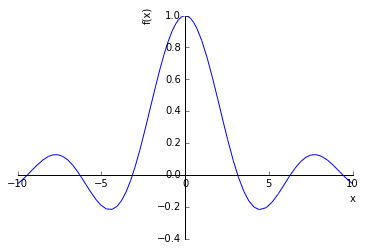Out:
<sympy.plotting.plot.Plot at 0xaf87e90c>

Several functions.

In :
plot(x,x**2,x**3,(x,0,2))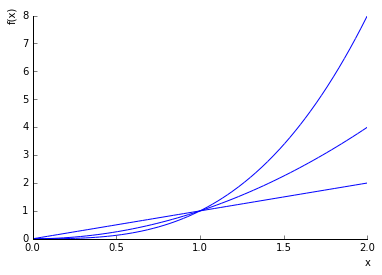Out:
<sympy.plotting.plot.Plot at 0xb136652c>

Some additional plotting functions can be imported from sympy.plotting.

In :
from sympy.plotting import (plot_parametric,plot_implicit,
plot3d,plot3d_parametric_line,
plot3d_parametric_surface)


A parametric plot - a Lissajous curve.

In :
t=Symbol('t')
plot_parametric(sin(2*t),cos(3*t),(t,0,2*pi),
title='Lissajous',xlabel='x',ylabel='y')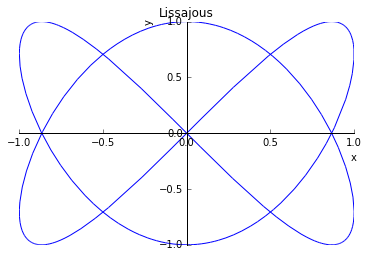Out:
<sympy.plotting.plot.Plot at 0xaf709d0c>

An implicit plot - a circle.

In :
plot_implicit(x**2+y**2-1,(x,-1,1),(y,-1,1))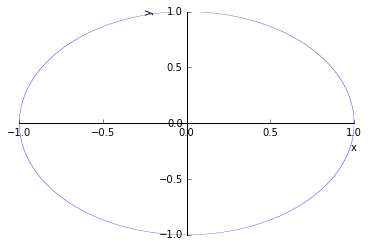Out:
<sympy.plotting.plot.Plot at 0xaf76e1ec>

A surface. If it is not inline but in a separaye window, you can rotate it with your mouse.

In :
plot3d(x*y,(x,-2,2),(y,-2,2))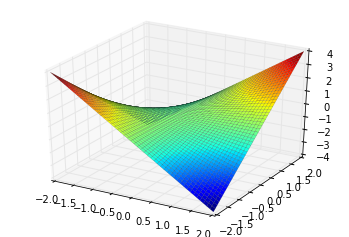Out:
<sympy.plotting.plot.Plot at 0xaf71ad2c>

Several surfaces.

In :
plot3d(x**2+y**2,x*y,(x,-2,2),(y,-2,2))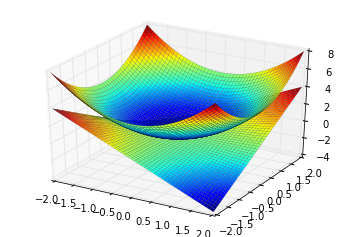Out:
<sympy.plotting.plot.Plot at 0xaf5a6f4c>

A parametric space curve - a spiral.

In :
a=0.1
plot3d_parametric_line(cos(t),sin(t),a*t,(t,0,4*pi))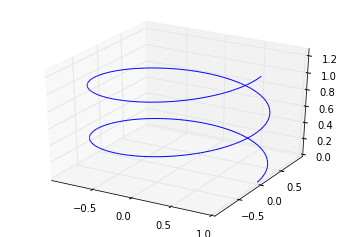Out:
<sympy.plotting.plot.Plot at 0xaf7d962c>

A parametric surface - a torus.

In :
u,v=symbols('u v')
a=0.3
plot3d_parametric_surface((1+a*cos(u))*cos(v),
(1+a*cos(u))*sin(v),a*sin(u),
(u,0,2*pi),(v,0,2*pi))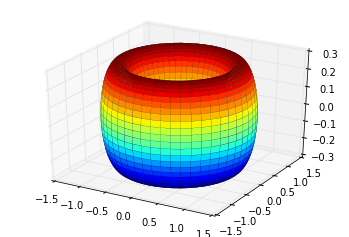Out:
<sympy.plotting.plot.Plot at 0xaf664e4c>